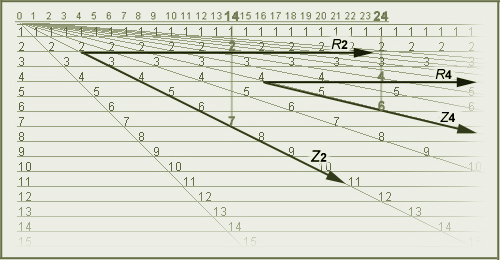Divisor Drips andSquare Root Waves Prime Numbers are the Holes in Complex Composite Number Patterns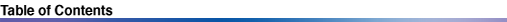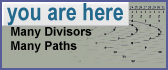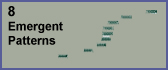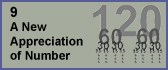7. Many Divisors, Many Paths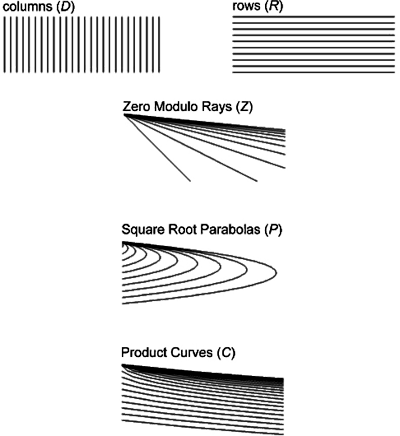I have identified five ways to classify divisors in the divisor plot. 1. Column Dx When considered as a member of a column, a divisor is simply one element of the set Dx. This is the classification which began this whole exploration. 2. Row (Ry) When considered as a member of a row Ry, a divisor is simply one of an infinite set of integers, which all have the same value - equal to its y coordinate. We have considered divisors along a row as representing a periodic signal over time, such as a blinking light or a beat in a musical polyrhythm. Differences in periods among rows are based on integers, and every possible integer period occurs in the set R. Seeing divisors as members of rows is one way to visualize the the Sieve or Eratosthenes. 3. Zero Reflection Ray (Zn) When considered as a member of a zero reflection ray, a divisor is one of an infinite, ordinal set of integers ocurring along one of the zero reflection rays Zn. 4. Square Root Parabola (Pn) When considered as a member of a square root parabola, a divisor is one of an ordinal set of integers from 0 to n, occurring on one of the square root parabolas Pn. 5. Product Curve (Cn) When considered as a member of a product curve, a divisor is one of an infinite, ordinal set of integers, occurring on one of the product curves Cn. The last three classifications are special: each consists of a set of curves, and each curve has an ordinal set of integers running through them. Let's take a good look at these last three classifications. Check out how each curve contains an ordinal set of integers. Zero Reflection Rays (Z)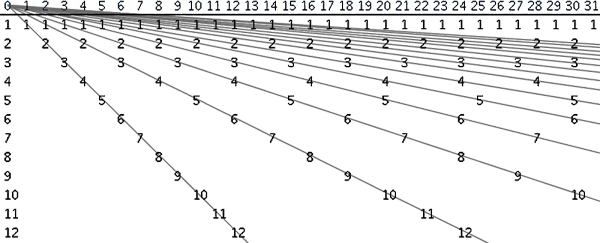Square Root Parabolas (P)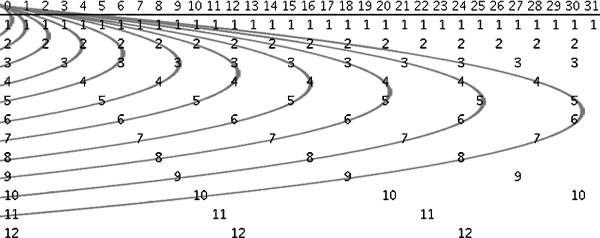Product Curves (C)Below we see that divisors occur where Z intersects with D: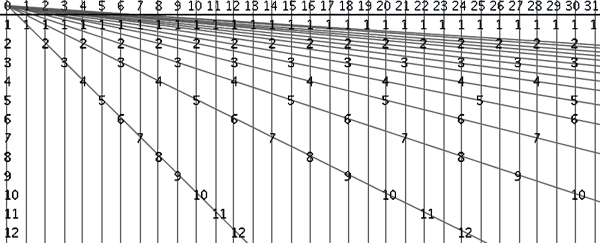And here we see that divisors occur where P intersects with D:Divisors also occur where P intersects with Z:And where P intersects with C: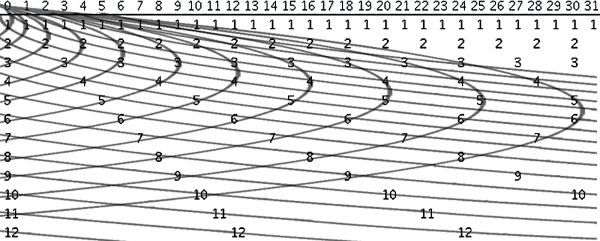And where Z intersects with C: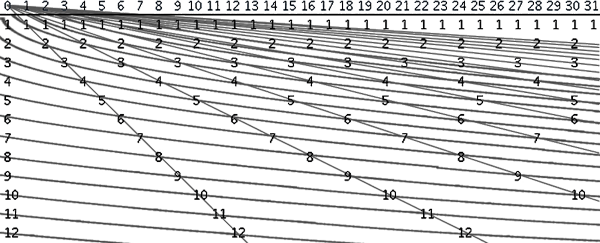This implies that divisors occur at the intersections of P, C and Z: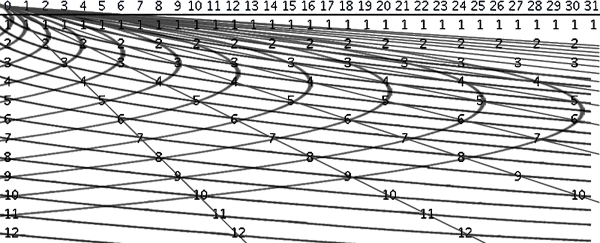Finally, we see all of these classifications overlaid. Note that all 5 sets of curves (D, R, Z, P, and C) intersect at divisors locations.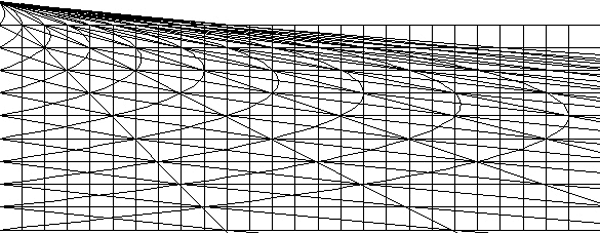Given these classifications, we now have three ways to define Dx (besides in terms of divisors): (1) Dx = the set of y values where x intersects P (2) Dx = the set of y values where x intersects Z (3) Dx = the set of y values where x intersects C Observe that for any n, Zn and Rn are associated. The illustration below shows that they each provide one of the divisors of a conjugate pair, whose product equals their x coordinate value. Two examples are illustrated: x = 14, and x = 24.